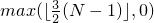# 25 Algorithms library [algorithms]

## 25.4 Sorting and related operations [alg.sorting]

### 25.4.7 Minimum and maximum [alg.min.max]

 template<class T> constexpr const T& min(const T& a, const T& b); template<class T, class Compare> constexpr const T& min(const T& a, const T& b, Compare comp); 

Requires: Type T is LessThanComparable (Table [lessthancomparable]).

Returns: The smaller value.

Remarks: Returns the first argument when the arguments are equivalent.

 template<class T> constexpr T min(initializer_list<T> t); template<class T, class Compare> constexpr T min(initializer_list<T> t, Compare comp); 

Requires: T is LessThanComparable and CopyConstructible and t.size() > 0.

Returns: The smallest value in the initializer_list.

Remarks: Returns a copy of the leftmost argument when several arguments are equivalent to the smallest.

 template<class T> constexpr const T& max(const T& a, const T& b); template<class T, class Compare> constexpr const T& max(const T& a, const T& b, Compare comp); 

Requires: Type T is LessThanComparable (Table [lessthancomparable]).

Returns: The larger value.

Remarks: Returns the first argument when the arguments are equivalent.

 template<class T> constexpr T max(initializer_list<T> t); template<class T, class Compare> constexpr T max(initializer_list<T> t, Compare comp); 

Requires: T is LessThanComparable and CopyConstructible and t.size() > 0.

Returns: The largest value in the initializer_list.

Remarks: Returns a copy of the leftmost argument when several arguments are equivalent to the largest.

 template<class T> constexpr pair<const T&, const T&> minmax(const T& a, const T& b); template<class T, class Compare> constexpr pair<const T&, const T&> minmax(const T& a, const T& b, Compare comp); 

Requires: Type T shall be LessThanComparable (Table [lessthancomparable]).

Returns: pair<const T&, const T&>(b, a) if b is smaller than a, and pair<const T&, const T&>(a, b) otherwise.

Remarks: Returns pair<const T&, const T&>(a, b) when the arguments are equivalent.

Complexity: Exactly one comparison.

 template<class T> constexpr pair<T, T> minmax(initializer_list<T> t); template<class T, class Compare> constexpr pair<T, T> minmax(initializer_list<T> t, Compare comp); 

Requires: T is LessThanComparable and CopyConstructible and t.size() > 0.

Returns: pair<T, T>(x, y), where x has the smallest and y has the largest value in the initializer list.

Remarks: x is a copy of the leftmost argument when several arguments are equivalent to the smallest. y is a copy of the rightmost argument when several arguments are equivalent to the largest.

Complexity: At most (3/2) * t.size() applications of the corresponding predicate.

 template<class ForwardIterator> ForwardIterator min_element(ForwardIterator first, ForwardIterator last); template<class ForwardIterator, class Compare> ForwardIterator min_element(ForwardIterator first, ForwardIterator last, Compare comp); 

Returns: The first iterator i in the range [first,last) such that for every iterator j in the range [first,last) the following corresponding conditions hold: !(*j < *i) or comp(*j, *i) == false. Returns last if first == last.

Complexity: Exactly max((last - first) - 1, 0) applications of the corresponding comparisons.

 template<class ForwardIterator> ForwardIterator max_element(ForwardIterator first, ForwardIterator last); template<class ForwardIterator, class Compare> ForwardIterator max_element(ForwardIterator first, ForwardIterator last, Compare comp); 

Returns: The first iterator i in the range [first,last) such that for every iterator j in the range [first,last) the following corresponding conditions hold: !(*i < *j) or comp(*i, *j) == false. Returns last if first == last.

Complexity: Exactly max((last - first) - 1, 0) applications of the corresponding comparisons.

 template<class ForwardIterator> pair<ForwardIterator, ForwardIterator> minmax_element(ForwardIterator first, ForwardIterator last); template<class ForwardIterator, class Compare> pair<ForwardIterator, ForwardIterator> minmax_element(ForwardIterator first, ForwardIterator last, Compare comp); 

Returns: make_pair(first, first) if [first,last) is empty, otherwise make_pair(m, M), where m is the first iterator in [first,last) such that no iterator in the range refers to a smaller element, and where M is the last iterator in [first,last) such that no iterator in the range refers to a larger element.

Complexity: At mostapplications of the corresponding predicate, where N is distance(first, last).